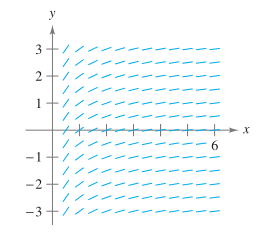Chapter 6.1, Problem 65E

Chapter
Section
Textbook Problem

Slope Field Use the slope field for the differential equation y ′ = 1 / x , where x > 0 to sketch thegraph of the solution that satisfies each given initial condition. Then make a conjecture about thebehavior of a particular solution of y ′ = 1 / x as x → ∞ . To print an enlarged copy of the graph, goto MathGraphs.com.a). ( 1 , 0 ) b). ( 2 , − 1 )

a)

To determine

To Graph: The solution that satisfies the given initial condition and then make a conjecture about the behavior of a particular solution of y=1/x as x.

Explanation

Given: The differential equation y=1/x, where x>0 and the point (1,0). The slope field for the differential equationis represented by the graph.

Graph:

We start by creating a table showing the slopes at several points.

The table shown below is a smallsample.

The slopes at many other points should be calculated to get a representative slope field.

 x 0 0 1 1 2 2 3 3 4 5 y -1 1 -1 1 -1 1 -1 1 -1 1 y′=1x 1 1 0.5 0.5 0.33 0.33 0.25 0.2

Now, we draw line segments at the points with their respective slopes as shown below.

After the slope field is drawn, we start from the starting point and the line goes right towards the direction of the line segment

b)

To determine

To Graph: The solution that satisfies the given initial condition and then make a conjecture about the behavior of a particular solution of y=1/x as x.

Still sussing out bartleby?

Check out a sample textbook solution.

See a sample solution

The Solution to Your Study Problems

Bartleby provides explanations to thousands of textbook problems written by our experts, many with advanced degrees!

Get Started

Solve the equations in Exercises 112 for x (mentally, if possible). 7x+55=98

Finite Mathematics and Applied Calculus (MindTap Course List)

Symmetry Test the equation for symmetry. 110. y = x2 + | x |

Precalculus: Mathematics for Calculus (Standalone Book)

In Exercises 19-24, find the functions f + g, f g, fg, and fg. 22. f(x) =1x2+1; g(x) = 1x21

Applied Calculus for the Managerial, Life, and Social Sciences: A Brief Approach

In words, explain what is measured by SS, variance, and standard deviation.

Statistics for The Behavioral Sciences (MindTap Course List)

In problems 15-22, simplify by combining like terms. 18.

Mathematical Applications for the Management, Life, and Social Sciences

Find each product: (6n)(5n2m)

Elementary Technical Mathematics